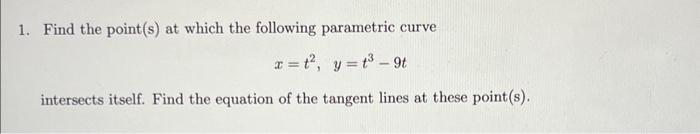# Question 1. Find the point(s) at which the following parametric curve $x=t^{2}, \quad y=t^{3}-9 t$ intersects itself. Find the equation of the tangent lines at these point(s).Transcribed Image Text: 1. Find the point(s) at which the following parametric curve $x=t^{2}, \quad y=t^{3}-9 t$ intersects itself. Find the equation of the tangent lines at these point(s).
Transcribed Image Text: 1. Find the point(s) at which the following parametric curve $x=t^{2}, \quad y=t^{3}-9 t$ intersects itself. Find the equation of the tangent lines at these point(s).&#12304;General guidance&#12305;The answer provided below has been developed in a clear step by step manner.Step1/3The equation of the parametric curve is $$\mathrm{{x}={t}^{{2}},{y}={t}^{{3}}-{9}{t}}$$now the point of intersection of this parametric curve itself is $$\mathrm{{y}={0}}$$ie \begin{align*} \mathrm{{t}^{{3}}-{9}{t}} &= \mathrm{{0}}\\[3pt]\mathrm{{t}{\left({t}^{{2}}-{9}\right)}} &= \mathrm{{0}}\\[3pt]\mathrm{{t}} &= \mathrm{{0}}\\[3pt]\mathrm{{t}^{{2}}} &= \mathrm{{9}}\\[3pt]\mathrm{{t}} &= \mathrm{\pm{3}} \end{align*}Explanation:point of self intersection is at a point on the x axis ie y=0Explanation:Please refer to solution in this step.Step2/3\begin{align*} \mathrm{{x}} &= \mathrm{{t}^{{2}}}\\[3pt]\mathrm{\frac{{\left.{d}{x}\right.}}{{\left.{d}{t}\right.}}} &= \mathrm{{2}{t}}\\[3pt]\mathrm{{y}} &= \mathrm{{t}^{{3}}-{9}{t}}\\[3pt]\mathrm{\frac{{\left.{d}{y}\right.}}{{\left.{d}{t}\right.}}} &= \mathrm{{3}{t}^{{2}}-{9}} \end{align*}now slope of the tangent ... See the full answer